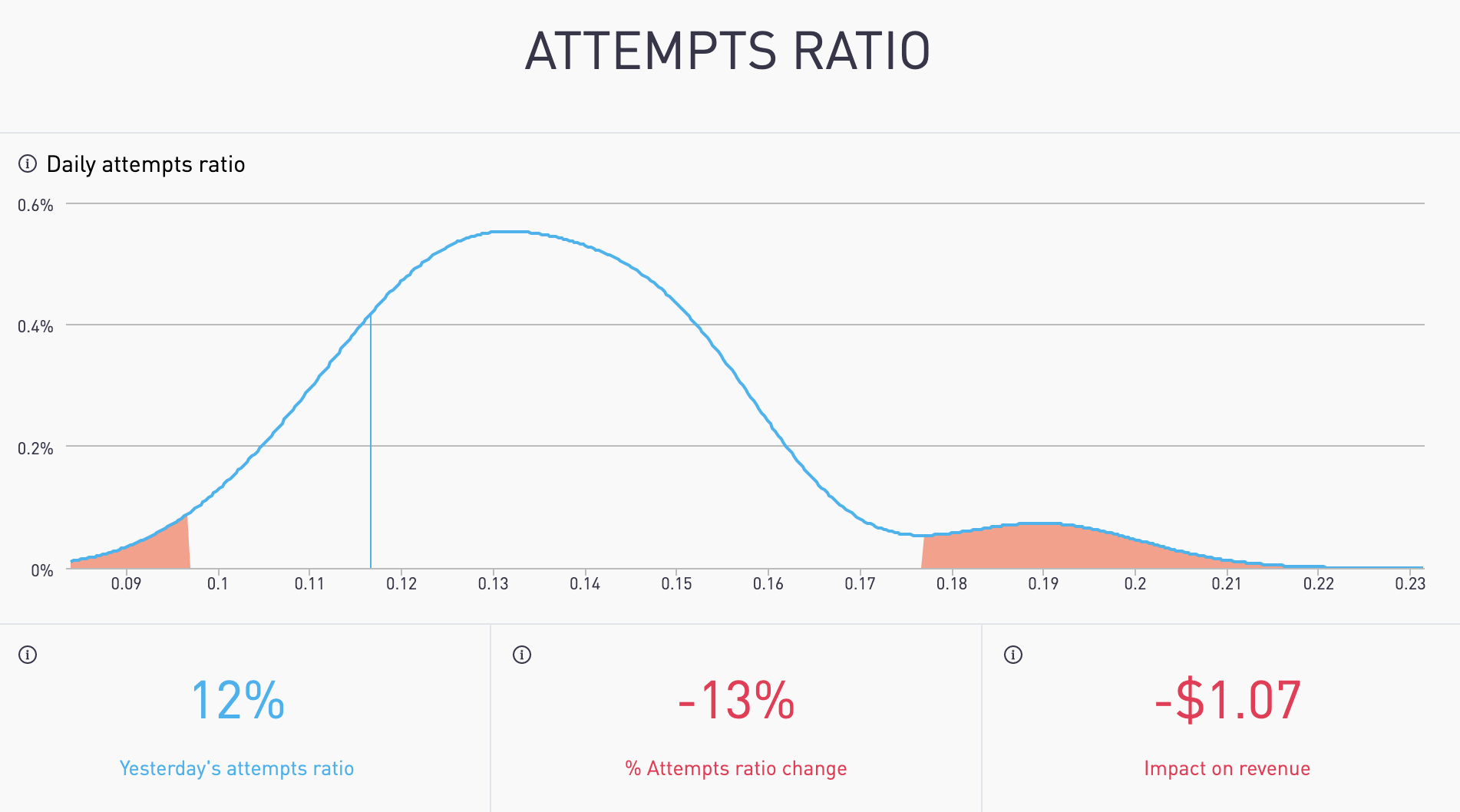# Attempts Ratio

How have the attempts ratio changed? What impact did it have on your daily revenue?## Is it common? Is it rare?

Density charts help you to see beyond simple averages to know if you need to take action. Decision-makers within companies have to focus on what matters. A simple average can't tell you when something is unusually good or bad. The density chart tells you how often something happens.

(density charts to detect trends) A density chart is the distribution of data over a continuous interval or time period.

# The Terms

Yesterday's Attempts Ratio

The total number of attempts divided by the number of shoppers for yesterday.

% Attempts Ratio Change

The change in yesterday's attempts ratio from the average of the last 4 weeks same day as yesterday attempts ratio as a percentage.

Impact on Revenue

The impact change in attempts ratio has on total revenue.

# The Math

Yesterday's Attempts Ratio

The total attempts divided by the total visitors to the payment page for yesterday.

% Attempts Ratio Change

If yesterday was "Sunday", then yesterday attempts ratio is compared with the average of previous 4 "Sunday's attempts ratio and represented as a percentage.

Impact on Revenue

Calculated by finding the regression coefficient for attempts ratio using linear regression multiplied with the change in attempts ratio(Eg-The difference between Sunday's attempts ratio & the previous 4 Sunday's attempts ratio multiplied with the regression coefficient), represented as a US dollar amount.

# The Visuals

X axis (or horizontal axis)

Attempts ratio

Y axis (or vertical axis)

How often the daily attempts ratio occurred during the period. This is also known as frequency.

Blue Curved Line

Each daily attempts ratio amount during the period shown on a smoothed line.

Blue Vertical Line

Yesterday's attempts ratio.